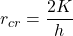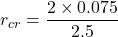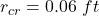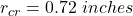## A 0.091-in-diameter electrical wire at 90°F is covered by 0.02-in-thick plastic insulation (k = 0.075 Btu/h·ft·°F). The wire is exposed to a

Question

A 0.091-in-diameter electrical wire at 90°F is covered by 0.02-in-thick plastic insulation (k = 0.075 Btu/h·ft·°F). The wire is exposed to a medium at 50°F, with a combined convection and radiation heat transfer coefficient of 2.5 Btu/h·ft2·°F. Calculate the critical radius (rcr) of the plastic insulation (in inches).

in progress 0
2 months 2021-07-22T12:24:59+00:00 1 Answers 4 views 0

The critical radius of the plastic insulation is 0.72 inches.

Explanation:

Given that,

Diameter = 0.091 in

Thickness = 0.02 in

Initial temperature = 90°F

Final temperature = 50°F

Heat transfer coefficient = 2.5 Btu/h.ft²°F

Material conductivity = 0.075 Btu/h.ft °F

We need to calculate the critical radius of the plastic insulationWhere, k = Material conductivity

h = Heat transfer coefficient

Put the value into the formulaHence, The critical radius of the plastic insulation is 0.72 inches.